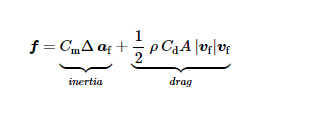# Morison Equation - Inertia and Cm

Hi Jason,

The Morison equation has two parts, the inertia part and the drag part.I understand that the drag part is calculated from the MemberCd values in Hydrodyn. How is the inertia part calculated ? Is my understanding correct here?

In the DeepCwind platform case I can find the Cd values from the hydrodyn input files. Where can I find the Cm values for the members?

Best regards,
Rahul.

Dear @Rahul.Ramachandran,

In the strip-theory formulation of HydroDyn for the force per unit length along each member (via Morison’s equation), HydroDyn uses Cm = Cp+Ca, where Cp represents the pressure coefficient (often taken as 1.0) for the Froude-Kriloff force and Ca represents the added-mass coefficient for the scattering force (also used in the calculation of added mass).

Best regards,

Dear Jason,

That’s clear. Thank you so much.

Rahul.